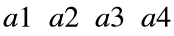# Problem 52030. Stacking vectors into a matrix

Given a 4x1 vector a, and a 2x2 matrix b, create a matrix M in which the first 2 rows are each identical to a and the last two rows correspond to two copies of the matrix b stacked next to each other.
In other words, if a = [] and b = [] , then
M = [].

### Solution Stats

69.39% Correct | 30.61% Incorrect
Last Solution submitted on Aug 04, 2023

### Community Treasure Hunt

Find the treasures in MATLAB Central and discover how the community can help you!

Start Hunting!